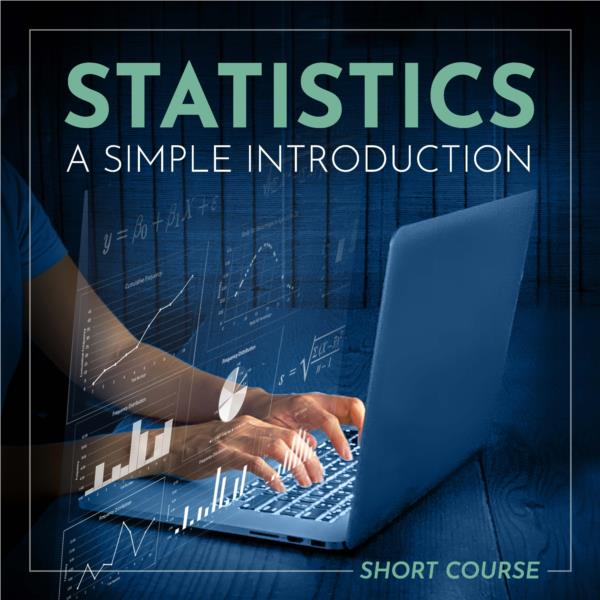# Statistics- A Simple Introduction- Short Course

Not Applicable

£100.00 Another currency?

 Tweet

# Introduction or refresher course covering the essentials of Statistics

If you need to learn or even just refresh your knowledge of statistics, this course is likely to be as simple a solution as you will find.  Statistics can be a rather dry subject for many, and yet it is something that has application in virtually every discipline.

Statistics informs us of trends, likely outcomes and possibilities that result from decisions taken and actions implemented. Without statistics, business decisions would be poorer, scientific discoveries would be fewer and resources would be managed less effectively both on macro and micro scales.

## Whats inside the 'Statistics- A Simple Introduction' Course?

LESSON 1 THE LANGUAGE
Example
Important Terms in Statistics
Data Collection Method
Statistical Method
Descriptive Statistics
Inferential Statistics
Data Point
Observation
Data Set
Research Population
Data Sample
Square Root
Exponents and Roots
Summation Sign
Array
Coefficient
Constant
Variable
Independent and Independent Variable
Univariate Data
Bivariate Data
Frequency Counts
Frequency Distribution
Grouped Frequency Table
Cumulative Frequency
Total Frequency
Bi-Variate Frequency
Class
Class Interval
Class Frequency
Data Types
Qualitative Data
Quantitative Data
Hypothesis
Responsible Use Of Statistics
The Use of Statistics in Everyday Life
More on Research Populations
The Use of Statistics By Governments and Organisations
Censuses
Educational Data
Developmental Data
Health Data
Economy
Marketing and Analytics
Review what you have been learning

LESSON 2 DATA COLLECTION
Tools for Data Collection
Collecting Data
Quantity
Timing
Time
Contamination/Bias
Language
Ethics
Cost
Source Reliability
Example
Sources of Data
Steps in Data Collection
Designing A Questionnaire
Review what you have been learning

LESSON 3 MEASURING THE CENTRE
Mode, Mean, Median
Mean
Mode
Median
Range
Standard Deviation
Normal Distribution
Which Measure Of Central Tendency To Use?
Box and Whisker Plots
Degrees Of Freedom
Standard Deviation
Review what you have been learning

LESSON 4 DETERMINING LIKELIHOODS (INFERENTIAL STATISTICS)
Measuring Probability
Probability Parameters
Discrete Variables
Continuous Variables
P-Values
Statistical Significance
Null and Alternate Hypothesis
Errors in Hypothesis Testing
Alpha
Beta
The T-Test
Types of T-Tests
A Review of Box and Whisker Plots Related to T-Tests
Analysis of Variance (ANOVA)
One-Way ANOVA
Parametric and Non-Parametric Tests
Review what you have been learning

LESSON 5 MEASURING CORRELATION
Correlation
Coefficient of Variation
Linear Regression
Simple Linear Regression Formula
Conclusion
Using Correlational Statistics
So why bother with correlations at all?
Statistics- A Simple Introduction- Short Course